## Insert Equations into the Live Editor

To describe a mathematical process or method used in your code, insert equations into your live script or function. Only text lines can contain equations. If you insert an equation into a code line, MATLAB® places the equation into a new text line directly under the selected code line.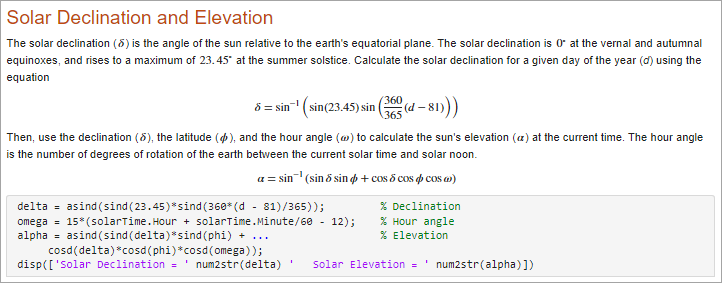There are two ways to insert an equation into a live script or function.

• Insert an equation interactively — You can build an equation interactively by selecting from a graphical display of symbols and structures.

• Insert a LaTeX equation — You can enter LaTeX commands and the Live Editor inserts the corresponding equation.

### Insert Equation Interactively

To insert an equation interactively:

1. Go to the Insert tab and click.

A blank equation appears.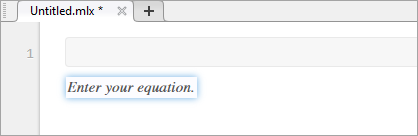2. Build your equation by selecting symbols, structures, and matrices from the options displayed in the Equation tab. View additional options by clicking the drop-down arrowto the right of each section.

When adding or editing a matrix, a context menu appears, which you can use to delete and insert rows and columns. You also can use the context menu to change or remove matrix delimiters.

3. Format your equation using the options available in the Text section. Formatting is only available for text within the equation. Numbers and symbols cannot be formatted. The formatting option is disabled unless the cursor is placed within text that can be formatted.

#### Keyboard Shortcuts for Equation Editing

The equation editor provides a few shortcuts for adding elements to your equation:

• To insert symbols, structures, and matrices, type a backslash followed by the name of the symbol. For example, type \pi to insert a π symbol into the equation. To discover the name of a symbol or structure, hover over the corresponding button in the Equation tab. You can also type backslash in the equation editor to bring up a completion menu of all supported names.Note

Although the \name syntax closely resembles LaTeX command syntax, entering full LaTeX expressions is not supported when inserting equations interactively.

• To insert subscripts, superscripts, and fractions, use the symbols ‘_’, ‘^’ or ‘/’. For example:

• Type x_2 to insert ${x}_{2}$ into the equation.

• Type x^2 to insert ${x}^{2}$ into the equation.

• Type x/2 to insert $\frac{x}{2}$ into the equation.

• To insert a new column into a matrix, type a ‘,’ at the end of the last cell in a matrix row. To insert a new row, type a semicolon ‘;’ at the end of the last cell in a matrix column.

• To insert the common symbols listed in this table, type a combination of other symbols.

Keyboard InputSymbolKeyboard InputSymbolKeyboard InputSymbol
| |=>!=
|=<-->!<
|–<->!>
–|<=!<=
->>=!>=
<-<>
<--~=

### Insert LaTeX Equation

To insert a LaTeX equation:

1. Go to the Insert tab, click, and select LaTeX Equation.

2. Enter a LaTeX expression in the dialog box that appears. For example, you can enter \sin(x) = \sum_{n=0}^{\infty}{\frac{(-1)^n x^{2n+1}}{(2n+1)!}}.

The preview pane shows a preview of equation as it would appear in the live script.3. To include a description of the LaTeX equation when exporting the live script to HTML, add text to the Alt Text field. For example, you can enter the text Maclaurin series for sin(x).

The description specifies alternative text for the equation and is saved as an alt attribute in the HTML document. It is used to provide additional information for the equation if, for example, a user is using a screen reader.

4. Press to insert the equation into your live script.

LaTeX expressions describe a wide range of equations. This table shows several examples of LaTeX expressions and their appearance when inserted into a live script.

LaTeX ExpressionEquation in Live Script
a^2 + b^2 = c^2${a}^{2}+{b}^{2}={c}^{2}$
\int_{0}^{2} x^2\sin(x) dx${\int }_{0}^{2}{x}^{2}\mathrm{sin}\left(x\right)dx$
\sin(x) = \sum_{n=0}^{\infty}{\frac{(-1)^n x^{2n+1}}{(2n+1)!}}$\mathrm{sin}\left(x\right)=\sum _{n=0}^{\infty }\frac{{\left(-1\right)}^{n}{x}^{2n+1}}{\left(2n+1\right)!}$
{a,b,c} \neq \{a,b,c\}$a,b,c\ne \left\{a,b,c\right\}$
x^{2} \geq 0\qquad \text{for all}x\in\mathbf{R}
\matrix{a & b \cr c & d}$\begin{array}{cc}a& b\\ c& d\end{array}$

### Supported LaTeX Commands

MATLAB supports most standard LaTeX math mode commands. These tables show a list of supported LaTeX commands.

#### Non-ASCII Letters

SymbolLaTeX CommandSymbolLaTeX CommandSymbolLaTeX Command
øoœoeåaa
ØOŒOEÅAA
łlæaeßss
ŁLÆAE

#### Greek/Hebrew Letters

SymbolLaTeX CommandSymbolLaTeX CommandSymbolLaTeX Command
αalphaνnuξxi
βbetaωomega ζzeta
χchiоomicronɛvarepsilon
δdeltaϕphiφvarphi
εepsilonπpi ϖvarpi
ηetaψpsiϱvarrho
vgamma ρrhoςvarsigma
ιiotaσsigma $\vartheta$vartheta
κkappaτtauאaleph
λlambda θtheta
μmuυupsilon
ΔDelta ΦPhiΘTheta
ΓGammaΠPi ϒUpsilon
ΛLambda ΨPsiΞXi
ΩOmega ΣSigma

#### Operator Symbols

SymbolLaTeX CommandSymbolLaTeX CommandSymbolLaTeX Command
*ast±pmwr
starmpcap
$·$cdot⨿amalgcup
circodot uplus
bulletominus sqcap
diamondoplus  sqcup
setminusoslash wedge, land
×timesotimesvee, lor
÷divbigoplustriangleleft
bot, perpbigotimestriangleright
topbigodot$△$bigtriangleup
prodbiguplus$▽$bigtriangledown
coprodbigcapbigvee
sumbigcupbigwedge
int, intopointbigsqcup
intbar

#### Relation Symbols

SymbolLaTeX CommandSymbolLaTeX CommandSymbolLaTeX Command
equiv <lt>gt
congle, leq ge, geq
neq, ne, not=not<not>
simprecsucc
simeqpreceqsucceq
approxllgg
asympsubsetsupset
doteqsubseteqsupseteq
proptosqsubseteqsqsupseteq
modelsmidin
bowtieparallelnotin
vdashiffni, owns
dashv

Note

Some commands can be combined with the not command to create the negated version of the symbol. For example, \not\leq creates the symbol . The commands that can be combined include leq, geq, equiv, cong, approx, sim, simeq, models, ni, parallel, succ, succeq, prec, preceq, subset, supset, subseteq, supseteq, sqsubseteq, and sqsupseteq.

#### Arrows

SymbolLaTeX CommandSymbolLaTeX CommandSymbolLaTeX Command
leftarrowrightarrowuparrow
LeftarrowRightarrowUparrow
longleftarrowlongrightarrowdownarrow
LongleftarrowLongrightarrowDownarrow
hookleftarrowhookrightarrowupdownarrow
leftharpoondownrightharpoondownUpdownarrow
leftharpoonuprightharpoonupleftrightarrow
swarrownearrowLeftrightarrow
nwarrowsearrowlongleftrightarrow
mapstolongmapstoLongleftrightarrow

#### Brackets

SymbolLaTeX CommandSymbolLaTeX CommandSymbolLaTeX Command
{lbrace}rbrace|vert
[lbrack]rbrackVert
langlerangle\backslash
lceilrceil
lfloorrfloor
llbracketrrbracket
SampleLaTeX CommandSampleLaTeX CommandSampleLaTeX Commandbig, bigl, bigr, bigm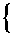bigg, biggl, biggr, biggm$\left\{abc\right\}$braceBig, Bigl, Bigr, BigmBigg, Biggl, Biggr, Biggm$\left[abc\right]$brack

#### Misc Symbols

SymbolLaTeX CommandSymbolLaTeX CommandSymbolLaTeX Command
infty forallwp
nablaexistsangle
partialemptysettriangle
Imıilozenge
Reȷjhbar
ellıimathprime
dots, ldots, hellipȷjmath¬lnot, neg
$\cdots$cdots:colon$\surd$surd
$\ddots$ddots$\cdot$cdotpgets
vdots$.$ldotpto
becausethereforevarpropto
°degreeclubsuitvarclubsuit
dag, daggerheartsuitvarheartsuit
ddag, ddaggerdiamondsuitvardiamondsuit
ldshspadesuitvarspadesuit
§mathsection

Note

The exists command can be combined with the not command to create the negated version of the symbol. For example, \not\exists creates the symbol ∄.

#### Text Symbols

SampleLaTeX CommandSampleLaTeX CommandSampleLaTeX Command
£pounds, textsterling¿textquestiondownquotedblbase
¢textcent¡textexclamdownbackprime
texteuro«flqq, guillemotleft space
¥yen»frqq, guillemotrightpermil
®textregistered§S\backslash
trademark, texttrademarkP¦brokenvert
©copyright

#### Accents

SymbolLaTeX CommandSymbolLaTeX CommandSymbolLaTeX Command
áacute$\stackrel{˙}{a}$dot$\stackrel{˜}{a}$tilde
$\overline{a}$bar$\stackrel{¨}{a}$ddot$\stackrel{\to }{a}$vec
$\stackrel{⌣}{a}$breve$\stackrel{⃛}{a}$dddotàgrave
$\stackrel{\text{ˇ}}{a}$check$\stackrel{⃜}{a}$ddddot$\stackrel{^}{a}$hat

#### Functions

SampleLaTeX CommandSampleLaTeX CommandSampleLaTeX Command
$\mathrm{arccos}$arccos$\mathrm{det}$det$\mathrm{ln}$ln
$\mathrm{arcsin}$arcsin$\mathrm{dim}$dim$\mathrm{log}$log
$\mathrm{arctan}$arctan$\mathrm{exp}$exp$\mathrm{max}$max
$\mathrm{arg}$arg$\mathrm{gcd}$gcd$\mathrm{min}$min
$\mathrm{cos}$cos$\mathrm{hom}$hom$\mathrm{Pr}$Pr
$\mathrm{cosh}$cosh$\mathrm{ker}$ker$\mathrm{sec}$sec
$\mathrm{cot}$cot$\mathrm{lg}$lg$\mathrm{sin}$sin
$\mathrm{coth}$coth$\mathrm{lim}$lim$\mathrm{sinh}$sinh
$\mathrm{csc}$csc$\mathrm{lim}\mathrm{inf}$liminf$\mathrm{sup}$sup
$\mathrm{deg}$deg$\mathrm{lim}\mathrm{sup}$limsup$\mathrm{tan}$tan

#### Math Constructs

SampleLaTeX CommandSampleLaTeX CommandSampleLaTeX Command
$\frac{abc}{xyz}$frac$\stackrel{a}{b}$stackrel, overset$\frac{a}{b}$over
$\sqrt{abc}$sqrt$\underset{a}{b}$underset$\left[\frac{a}{b}\right]$overwithdelims
$\text{\hspace{0.17em}}\mathrm{mod}\text{\hspace{0.17em}}a$bmod$\left(\begin{array}{c}a\\ b\end{array}\right)$binom, choose$\stackrel{←}{abc}$overleftarrow
$\text{\hspace{0.17em}}\text{\hspace{0.17em}}\text{\hspace{0.17em}}\text{ }\left(\mathrm{mod}a\right)$pmod$\left(\begin{array}{cc}a& b\\ c& d\end{array}\right)$pmatrix$\stackrel{\to }{abc}$overrightarrow
$\stackrel{^}{abc}$widehat$\begin{array}{cc}a& b\\ c& d\end{array}$matrix$\stackrel{↔}{abc}$overleftrightarrow
$\stackrel{˜}{abc}$widetilde$\begin{array}{cc}a& b\\ c& d\end{array}$begin{array}$\underset{¯}{\begin{array}{c}\overline{ab}\\ cd\end{array}}$hline
$〈abc|$bra$\left\{\begin{array}{ll}a\hfill & b\hfill \\ c\hfill & d\hfill \end{array}$begin{cases}$\sum _{i=0}^{10}$limits
$|abc〉$ket$\left\{\frac{a}{2}|{b}^{2}\right\}$left, middle, right${\sum }_{i=0}^{10}$nolimits
$〈ab|cd〉$braket

Note

To create a matrix using the matrix and pmatrix commands, use the & symbol to separate columns, and \cr to separate rows. For example, to create a 2–by–2 matrix, use the expression \matrix{a & b \cr c & d}.

For large operators, MATLAB automatically adjusts the position of limits depending on whether or not there is text inline with the equation. To force the display of the limits above and below the operator, use the limits command. To force the display of the limits adjacent to the operator, use the \nolimits command. For example, the expression \sum\limits_{i=0}^{10} displays the limits of the summation operator above and below the operator. The expression \sum\nolimits_{i=0}^{10} displays the limits of the summation operator adjacent to the operator.

$\sum _{i=0}^{10}$${\sum }_{i=0}^{10}$

#### White Space

SampleLaTeX CommandSampleLaTeX CommandSampleLaTeX Command
$ab$negthinspace$abc$mathord$a\left[b$mathopen
$ab$thinspace$a\sum b$mathop$a\right]b$mathclose
$a\text{\hspace{0.17em}}b$enspace$a+b$mathbin$a\text{\hspace{0.17em}}\text{\hspace{0.17em}}|\text{ }\text{\hspace{0.17em}}\text{\hspace{0.17em}}b$mathinner
$a\text{\hspace{0.17em}}\text{\hspace{0.17em}}\text{\hspace{0.17em}}\text{ }b$quad$a=b$mathrel$a\text{\hspace{0.17em}}\text{\hspace{0.17em}}\text{\hspace{0.17em}}\text{ }b$kern, mkern
$a\text{ }\text{\hspace{0.17em}}\text{\hspace{0.17em}}\text{\hspace{0.17em}}b$qquad$a,b$mathpunct

#### Text Styling

SampleLaTeX CommandSampleLaTeX CommandSampleLaTeX Commanddisplaystyle$\text{ABCDE}$text, textnormal$\text{ABCDE}$texttt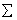textstyle$ABCDE$bf, textbf, mathbf$ABCDE$textsf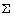scriptstyle$ABCDE$it, textit, mathit$\mathcal{A}ℬ\mathcal{C}\mathcal{D}ℰ$cal, mathcalscriptscriptstyle$\text{ABCDE}$rm, textrm, mathrm$\mathbb{A}\mathbb{B}ℂ\mathbb{D}$mathbb
$\text{ABCDE}$hbox, mbox$\mathfrak{A}\mathfrak{B}ℭ\mathfrak{D}$mathfrak